Jekyll2022-01-14T23:36:03+01:00https://royfrancis.com/feed.xmlrmfCode, Photography, DesignRoy FrancisExploring history and culture in Thessaloniki2019-11-07T00:00:00+01:002019-11-07T00:00:00+01:00https://royfrancis.com/greece-thessalonikiI took a short autumn trip to Thessaloniki, Greece.

This place is most interesting for it’s culture and history. You can learn all about the Greek civilisation and the evolution of human civilisation in general. Some of my favourite musuems were the Archaeological Museum of Thessaloniki, War Museum of Thessaloniki and the white tower museum. The Museum of Byzantine Culture was unfortunately closed. There are numerous historical churches and archaeological sites scattered through the city.

There are a few places to get some great views of the city. The white tower museum is the most obvious, but it is not that high. The skyline cafe bar offers excellent 360 degree views of the city as you stuff your pie-hole. Another site is the Trigonion Tower which offers stunning views over the whole city.

On the last day, I took a train ride down to Platamon which is about 1.5 hrs away from Thessaloniki. The beaches were practically empty in November and to be fair, it wasn’t very warm to be in the water. But, I had an excellent fried fish dinner at a local restaurant.

]]>
Roy Francis
Scatterplot annotation2019-09-17T00:00:00+02:002019-09-17T00:00:00+02:00https://royfrancis.com/scatterplot-annotationIn this post, we are exploring ideas to mark clusters of points on a scatterplot for labelling purposes. Or in other words, how to draw polygons around scatterplots. We use ggplot2 for plotting and few different functions to generate the markings. The required packages are shown below. A custom ggplot2 theme is used to simplify the plot.

library(dplyr)
library(ggplot2)
library(ggforce)
library(ggalt)
data(iris)

theme_custom <- function (basesize=14) {
theme_bw(base_size=basesize) %+replace%
theme(
panel.border=element_blank(),
panel.grid=element_blank(),
legend.position="top",
legend.direction="horizontal",
legend.justification="right",
strip.background=element_blank(),
axis.ticks=element_blank(),
axis.title=element_blank(),
axis.text=element_blank()
)
}

We are using the iris dataset using the three different species as groups/clusters.

iris %>%
ggplot(aes(Petal.Length, Petal.Width, colour=Species))+
geom_point()+
theme_custom()

Base

First we start by using base functions.

Ellipse

Perhaps the easiest approach is drawing confidence ellipse. All points are not guaranteed to be inside the ellipse. The default level is 0.95. Increasing this includes more of the points, but the ellipses may get too large. The nice thing about this approach is that it is not strongly affected by outliers. This is well suited for clusters where points have a large spread and overlap a lot.

ggplot(data=iris, aes(Petal.Length, Petal.Width, colour=Species))+
geom_point()+
stat_ellipse(level=0.95)+
theme_custom()

For most part, this is the easiest approach and good enough.

Convex hull

The outermost points in a point cloud can be connected to form a polygon referred to as the convex hull of a point cloud. The convex hull can be computed using the base function chull(). This can be used in a dplyr pipeline as shown below.

hull <- iris %>%
group_by(Species) %>%
slice(c(chull(Petal.Length, Petal.Width),
chull(Petal.Length, Petal.Width)))

The information to draw polygons around all three clusters is contained in the data.frame hull. The points and the polygons can be plotted as shown below.

ggplot()+
geom_point(data=iris, aes(Petal.Length, Petal.Width, colour=Species))+
geom_path(data=hull, aes(Petal.Length, Petal.Width, colour=Species))+
theme_custom()

This is a good start, but it would be nicer if the edges of the polygon had smooth edges rather than sharp edges. While we could use some custom script to smooth it, the easier approach is to use from existing packages.

Density and contours

Before we move on, another idea from the base functions is to compute a 2D kernal density from the point cloud and draw countour lines.

iris %>%
ggplot(aes(Petal.Length, Petal.Width, colour=Species))+
geom_point()+
geom_density_2d()+
theme_custom()

ggalt

geom_encircle

We use the function geom_encircle() from package ggalt to draw smoothed lines around clusters.

ggplot(data=iris, aes(Petal.Length, Petal.Width, colour=Species))+
geom_point()+
ggalt::geom_encircle(size=1.6)+
theme_custom()

ggforce

Another useful package for marking clusters is ggforce. Below is a demonstration of four functions for marking clusters. The four functions are geom_mark_rect(), geom_mark_circle(), geom_mark_ellipse() and geom_mark_hull(). The shapes they create is self-explanatory.

p1 <- ggplot(data=iris, aes(Petal.Length, Petal.Width, colour=Species))+
geom_point()+
geom_mark_rect()+
theme_custom()

p2 <- ggplot(data=iris, aes(Petal.Length, Petal.Width, colour=Species))+
geom_point()+
geom_mark_circle()+
theme_custom()

p3 <- ggplot(data=iris, aes(Petal.Length, Petal.Width, colour=Species))+
geom_point()+
geom_mark_ellipse()+
theme_custom()

p4 <- ggplot(data=iris, aes(Petal.Length, Petal.Width, colour=Species))+
geom_point()+
geom_mark_hull()+
theme_custom()

ggpubr::ggarrange(p1, p2, p3, p4, nrow=2, ncol=2, common.legend=T)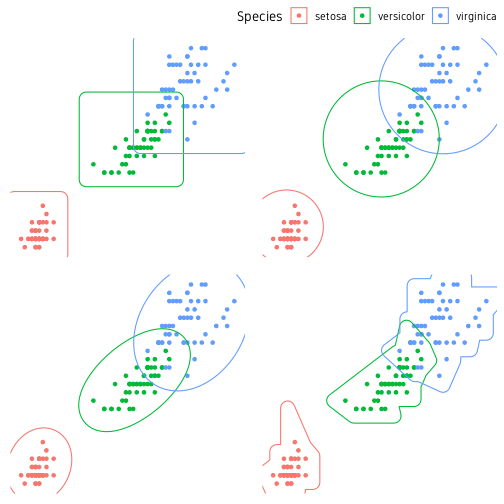Fig. 5: Clusters demarcated using rectangles (top-left), circles (top-right), ellipses (bottom-left) and smoothed convex hull (bottom-right).

geom_mark_hull() has extra arguments to control concavity, radius and size (expand) of the hull shape. geom_mark_* functions also support an argument label to label the the marks. In this case, the plot legend can be turned off.

ggplot(data=iris, aes(Petal.Length, Petal.Width, colour=Species))+
geom_point()+
geom_mark_ellipse(aes(label=Species), label.colour="grey30")+
theme_custom()+
theme(legend.position="none")

It is not necessary to mark all the groups. A particular group of interest can be marked alone.

ggplot()+
geom_point(data=iris, aes(Petal.Length, Petal.Width))+
theme_custom()+
theme(legend.position="none")

For more control over text annotation, see package ggrepel.

The ggforce package also has a function called geom_shape() to draw polygons but with some neat features. We can adjust the corner radius of the polygon to smooth the edges. We can also increase or decrease the overall size of the polygon. In this case, we use the convex hull object hull which we computed earlier.

p1 <- ggplot()+
geom_point(data=iris, aes(Petal.Length, Petal.Width, colour=Species))+
geom_shape(data=hull, aes(Petal.Length, Petal.Width, colour=Species), fill=NA)+
theme_custom()
p2 <- ggplot()+
geom_point(data=iris, aes(Petal.Length, Petal.Width, colour=Species))+
geom_shape(data=hull, aes(Petal.Length, Petal.Width, colour=Species), fill=NA, radius=0.05)+
theme_custom()
p3 <- ggplot()+
geom_point(data=iris, aes(Petal.Length, Petal.Width, colour=Species))+
geom_shape(data=hull, aes(Petal.Length, Petal.Width, colour=Species), fill=NA, radius=0.05, expand=0.04)+
theme_custom()
ggpubr::ggarrange(p1, p2, p3, nrow=2, ncol=2, common.legend=T)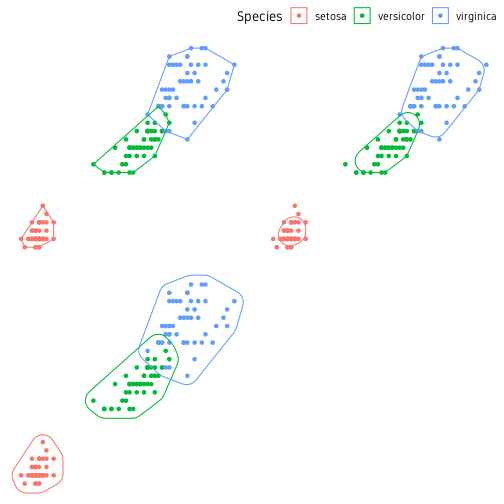Fig. 8: Clusters marked using manually computed convex hull. geom_shape from ggforce is used. Standard convex hull (top-left), smoothed edges (top-right) and smoothed+enlarged (bottom-left).

The package ggforce has many more cool features. You should check out their website.

]]>
Roy Francis
Exploring the Swedish west coast2019-08-22T00:00:00+02:002019-08-22T00:00:00+02:00https://royfrancis.com/sweden-west-coast

The west coast of Sweden is well known for it’s rocky archipelago and scenic beauty. Also known for seafood, sea kayaking and floating saunas. I took a trip through Lidköping and Trollhättan to Lysekil, Fjällbacka and Grebbestad.

In Trollhättan, the Saab car museum, Trollhättan canal and Olidan power station were interesting. About 30 mins north of Lysekil is the animal park Nordens ark. It’s a fun place if you are into animals.

I slowly drove around between Lysekil and Grebbestad stopping at every scenic spot. There are also many camping spots around the area which makes it convenient. I stayed at Fjällbacka camping for one of the nights was a really cute campsite with glorious views over the archipelago.

]]>
Roy Francis
Road trip through Portugal2019-06-30T00:00:00+02:002019-06-30T00:00:00+02:00https://royfrancis.com/portugalI attended the Elixir hands-on meeting in Lisbon, Portugal this summer. This gave me an opportunity to visit Lisbon and take a road trip through Portugal.

I had never been to Lisbon before, so I wasn’t exactly sure what to expect. But, Lisbon was absolutely lovely. The weather was not too hot and the cute winding streets were charming and bustling with people. Parts of the city are hilly and scenic viewpoints are everywhere. The city is full of historic buildings, museums, restaurants and cafes. I thought the Maritime museum was very interesting. The food was reasonably priced and delicious. I also happened to be in perfect time for the Lisbon street festivals which meant that there were parties and dancing in the streets almost all through the city.

After the conference, I rented a car and drove down South to Algarve exploring the countryside.

There are several beautiful sandy beaches along the west coast as well as the South. Few of them that I visited were Praia da Zambujeira do Mar, Praia de Odeceixe, Praia da Cordoama and Praia do Zavial.

Cape St. Vincent (Farol do Cabo de São Vicente) is the Southwestern tip of Portugal, which also happens to be the most Southwestern point of continental Europe. The lighthouse and rocky cliffs are magnificent. I arrived here in the evening just before sunset. The golden hour was amazing and then out of nowhere, the fog came in. The heavy fog drifting across the cliffs and over the peninsula was an amazing sight from the drone.

The coastal areas around Lagos, Portimão and Faro have rather interesting rock formations and cave systems. Some of them are Benagil caves (Algar de Benagil), Marinha beach (Praia da Marinha), Arco de Albandeira and Algar Seco. I stayed in Albufeira for a couple of days to check out the attractions. I also went on a dolphin watching trip which was exciting.

If you are into pirates, you should check out the Pirate shop in Sagres and the Pirate themed B&B in Charneca de Caparica.

]]>
Roy Francis
A guide to elegant tiled heatmaps in R 2019-02-17T23:07:48+01:002019-02-17T23:07:48+01:00https://royfrancis.com/a-guide-to-elegant-tiled-heatmaps-in-r-2019This is an update to an older post from 2015 on the same topic. This covers the exact same thing but using the latest R packages and coding style using the pipes (%>% ) and tidyverse packages.

This was inspired by the disease incidence rate in the US featured on the Wall Street Journal. The disease incidence dataset was originally used in this article in the New England Journal of Medicine. In this post, I am using the measles level 1 incidence (cases per 100,000 people) dataset obtained as a .csv file from Project Tycho. Download the .csv file here.

In this post, we will look into creating a neat, clean and elegant heatmap in R. No clustering, no dendrograms, no trace  lines, no bullshit. We will go through some basic data cleanup, reformatting and finally plotting. We go through this step by step. For the whole code with minimal explanations, scroll to the bottom of the page.

I am running R version 3.5.2 64-bit on Ubuntu 18.04 64-bit. Packages in use are ggplot2 (3.1.0), dplyr (0.7.8), tidyr (0.8.2), stringr (1.3.1) and for base plots I am using gplots (3.0.1.1) and plotrix (3.7-4). Install the necessary packages if not already installed and load them.

# install packages
# install.packages(pkgs = c("ggplot2","dplyr","tidyr","stringr","gplots","plotrix"),dependencies = T)

library(ggplot2) # ggplot() for plotting
library(dplyr) # data reformatting
library(tidyr) # data reformatting
library(stringr) # string manipulation

1. Data preparation

Read in the .csv file and inspect the data. The first two rows with non-table data is skipped.

# inspect data
str(m)
table(m\$YEAR)
table(m\$WEEK)

The head() function shows us the header names and the first 6 rows of the data. The str() function shows that YEAR and WEEK columns are stored as integers and the incidences as characters. The incidences although numeric have been read in as character because missing values are coded as “-“.  The table() function is used to check for any missing years or weeks. The data is currently stored in so called ‘wide’ format which we will convert to ‘long’ format. The ggplot2 plotting package prefers long format. The wide format is shown below.

1 1928    1    3.67      -    1.90     4.11       1.38     8.38
2 1928    2    6.25      -    6.40     9.91       1.80     6.02
3 1928    3    7.95      -    4.50    11.15       1.31     2.86
4 1928    4   12.58      -    1.90    13.75       1.87    13.71
5 1928    5    8.03      -    0.47    20.79       2.38     5.13
6 1928    6    7.27      -    6.40    26.58       2.79     8.09

The YEARand WEEK variables are kept as is and all incidence values are collapsed into a variable and value column. The column names are changed to lower case for convenience. The year and week variables are converted to factors and value variable is converted to a number.

m2 <- m %>%
# convert data to long format
gather(key="state", value="value", -YEAR, -WEEK) %>%
# rename columns
setNames(c("year", "week", "state", "value")) %>%
# convert year to factor
mutate(year=factor(year)) %>%
# convert week to factor
mutate(week=factor(week)) %>%
# convert value to numeric (also converts '-' to NA, gives a warning)
mutate(value=as.numeric(value))

The output in long format is shown below.

year week   state value
1 1928    1 Alabama  3.67
2 1928    2 Alabama  6.25
3 1928    3 Alabama  7.95
4 1928    4 Alabama 12.58
5 1928    5 Alabama  8.03
6 1928    6 Alabama  7.27

The state variable is currently in all caps and multi-word states have dot separators. I prefer to have them in Title Case and separated by space to be shown on the plot later. A custom function is used to change the states into Title Case. Multi-word states are split at the dot separator and each word is changed into Title Case using the function str_to_title() and they are pasted back together.

# removes . and change states to title case using custom function
fn_tc <- function(x) paste(str_to_title(unlist(strsplit(x, "[.]"))), collapse=" ")
m2\$state <- sapply(m2\$state, fn_tc)

Now, I would like to plot the heatmap with the year on the x-axis and state on the y-axis, which means we have to deal with the week variable in some way. We will sum all the incidences from all weeks for each year and discard the week variable. The dplyr compliant way to do this is to use group_by() followed by summarise() using a function.

The way sum() handles NAs is a bit strange. By default, it returns NA if one or more elements in the input vector is NA. If we set argument na.rm=TRUE, then NAs are removed and the remaining numbers are summed. But if all the elements are NA, the sum is returned as zero. This is weird and undesirable in this situation. Therefore, I have a custom sum function named na_sum() to remove NAs and return the remaining sum or return NA if all elements are NA. We then use this custom function inside summarise() function to summarise the data by year and state while getting rid of week.

# custom sum function returns NA when all values in set are NA,
# in a set mixed with NAs, NAs are removed and remaining summed.
na_sum <- function(x)
{
if(all(is.na(x))) val <- sum(x, na.rm=F)
if(!all(is.na(x))) val <- sum(x, na.rm=T)
return(val)
}

# sum incidences for all weeks into one year
m3 <- m2 %>%
group_by(year, state) %>%
summarise(count=na_sum(value)) %>%
as.data.frame()

Now our data looks like this without the week variable. The values are summed by year for each state to create a new variable count.

year      state  count
1 1928    Alabama 334.99
3 1928    Arizona 200.75
4 1928   Arkansas 481.77
5 1928 California  69.22

At this point, the data preparation is essentially over. The data is in ‘long’ format, the x, y and z variables for the plot are available and are of the correct type: factor, factor and numeric. If your data is already in this format, it is easy to jump straight into visualisation. But depending on what sort of data you start off with, the data preparation and reformatting can be complicated and tedious.

2. Plotting

I prefer to use the ggplot2 plotting package to plot graphs in R due to its consistent code structure. I will focus mostly on ggplot2 code here. But, just for the sake of completeness, I will also include some heatmap code using base graphics.

2.1 ggplot2

Once the data is in the right format, plotting the data is rather simple code in ggplot2. The ggplot2 index page has the code syntax and arguments.

#basic ggplot
p <- ggplot(m3, aes(x=year, y=state, fill=count))+
geom_tile()

#save plot to working directory
ggsave(p, filename="measles-basic.png")

The default output from ggplot2 is quite decent- There are several aspects of the basic plot that can be modified and tweaked. We add tile borders, custom x-axis breaks and custom text sizes. The ggplot code is modified below.

#modified ggplot
p <- ggplot(m3, aes(x=year, y=state, fill=count))+
#add border white colour of line thickness 0.25
geom_tile(colour="white", size=0.25)+
#remove x and y axis labels
labs(x="", y="")+
#remove extra space
scale_y_discrete(expand=c(0, 0))+
#define new breaks on x-axis
scale_x_discrete(expand=c(0, 0),
breaks=c("1930", "1940", "1950", "1960", "1970", "1980", "1990", "2000"))+
#set a base size for all fonts
theme_grey(base_size=8)+
#theme options
theme(
#bold font for legend text
legend.text=element_text(face="bold"),
#set thickness of axis ticks
axis.ticks=element_line(size=0.4),
#remove plot background
plot.background=element_blank(),
#remove plot border
panel.border=element_blank()
)

#save with dpi 200
ggsave(p, filename="measles-mod1.png", height=5.5, width=8.8, units="in", dpi=200)

I would prefer the y-axis labels (states) to be ordered ascending top-bottom. This means going back to our ‘long’ format data and refactoring the state variable in reverse. The fill variable here (count) is a continuous variable and hence, ggplot by default uses the blue colour ramp. Here and in many cases, it might make better sense to bin the continuous data into levels and represent each level as a discrete colour. The cut() function in R allows to break and label a continuous variable.

The count variable is binned into 7 levels and saved as a new variable countfactor. The NAs remain as NA. Defining breaks in your variable depends on the type of data, the number of bins that make sense with the context or just trial and error. Too many bins are not good. Checking your variable using summary(x) or a boxplot(x) can reveal a lot about the data.

m4 <- m3 %>%
# convert state to factor and reverse order of levels
mutate(state=factor(state, levels=rev(sort(unique(state))))) %>%
# create a new variable from count
mutate(countfactor=cut(count, breaks=c(-1, 0, 1, 10, 100, 500, 1000, max(count, na.rm=T)),
labels=c("0", "0-1", "1-10", "10-100", "100-500", "500-1000", ">1000"))) %>%
# change level order
mutate(countfactor=factor(as.character(countfactor), levels=rev(levels(countfactor))))

Now we are ready to plot the final dataset.

# assign text colour
textcol <- "grey40"

# further modified ggplot
p <- ggplot(m4, aes(x=year, y=state, fill=countfactor))+
geom_tile(colour="white", size=0.2)+
guides(fill=guide_legend(title="Cases per\n100,000 people"))+
labs(x="", y="", title="Incidence of Measles in the US")+
scale_y_discrete(expand=c(0, 0))+
scale_x_discrete(expand=c(0, 0), breaks=c("1930", "1940", "1950", "1960", "1970", "1980", "1990", "2000"))+
scale_fill_manual(values=c("#d53e4f", "#f46d43", "#fdae61", "#fee08b", "#e6f598", "#abdda4", "#ddf1da"), na.value = "grey90")+
#coord_fixed()+
theme_grey(base_size=10)+
theme(legend.position="right", legend.direction="vertical",
legend.title=element_text(colour=textcol),
legend.margin=margin(grid::unit(0, "cm")),
legend.text=element_text(colour=textcol, size=7, face="bold"),
legend.key.height=grid::unit(0.8, "cm"),
legend.key.width=grid::unit(0.2, "cm"),
axis.text.x=element_text(size=10, colour=textcol),
axis.text.y=element_text(vjust=0.2, colour=textcol),
axis.ticks=element_line(size=0.4),
plot.background=element_blank(),
panel.border=element_blank(),
plot.margin=margin(0.7, 0.4, 0.1, 0.2, "cm"),
plot.title=element_text(colour=textcol, hjust=0, size=14, face="bold")
)

#export figure
ggsave(p, filename="measles-mod3.png", height=5.5, width=8.8, units="in", dpi=200)

This is the final version of the figure. All font elements are coloured grey40.  Missing values (NAs) are coloured grey90. The year of the introduction of vaccination is indicated as a dark vertical stripe. If the y-axis labels are too many or too small, they can be dropped the same way as the x-axis. A custom colour palette was used from ColorBrewer based on the Spectral palette. Using the R package RColorBrewer() or using ggplot2 function scale_fill_brewer() opens up all the colour palettes from the ColorBrewer website. Here is an example below:

library(RColorBrewer)

#change the scale_fill_manual from previous code to below
scale_fill_manual(values=rev(brewer.pal(7, "YlGnBu")), na.value="grey90")+

Most of the plot customisation happens in the theme() section of the code. Refer to the ggplot index for all theme arguments. Based on where the figure will go next, the fonts may need to be changed. The extrafont() package comes handy. Another option is to export in vector format such as .svg or .pdf. Import into a vector editor such as Adobe Illustrator and add your own text. This will produce nice crisp results but does take some manual work. See the cover image of this post for example.

2.2 Base graphics

We will take a quick look at plotting using base graphics. The base function heatmap() and the enchanced heatmap.2() function from the gplots package uses a wide format data matrix as input. We start with the ‘long’ data that we prepared in section 1. We convert the data in ‘long’ format into ‘wide’ format using the spread() function from package tidyr. The wide data is converted to a matrix after removal of non-numeric columns. The state names are reassigned as rownames of the matrix to be used as y-axis text.

library(gplots) # heatmap.2() function

# convert from long format to wide format
m5 <- m3 %>% spread(key="state", value=count)
m6 <- as.matrix(m5[ ,-1])
rownames(m6) <- m5\$year

#base heatmap
png(filename="measles-base.png", height=5.5, width=8.8, res=200, units="in")
heatmap(t(m6), Rowv=NA, Colv=NA, na.rm=T, scale="none", col=terrain.colors(100),
xlab="", ylab="", main="Incidence of Measles in the US")
dev.off()

That’s pretty much what we can do with that. The enchanced heatmap.2() function from gplots package can do more. The legend for the colours is not the best, so we will use the function gradient.rect() from plotrix package to create our own legend. And after fiddling around with numerous arguments and countless trial and errors later, we have as below.

# gplots heatmap.2
png(filename="measles-gplot.png", height=6, width=9, res=200, units="in")
par(mar=c(2, 3, 3, 2))
gplots::heatmap.2(t(m6), na.rm=T, dendrogram="none", Rowv=NULL, Colv="Rowv", trace="none", scale="none", offsetRow=0.3, offsetCol=0.3, breaks=c(-1, 0, 1, 10, 100, 500, 1000, max(m4\$count, na.rm=T)), colsep=which(seq(1928, 2003)%%10==0), margin=c(3, 8), col=rev(c("#d53e4f", "#f46d43", "#fdae61", "#fee08b", "#e6f598", "#abdda4", "#ddf1da")),
xlab="", ylab="", key=F, lhei=c(0.1, 0.9), lwid=c(0.2, 0.8))
gradient.rect(0.125, 0.25, 0.135, 0.75, nslices=7, border=F, gradient="y", col=rev(c("#d53e4f", "#f46d43", "#fdae61", "#fee08b", "#e6f598", "#abdda4", "#ddf1da")))
text(x=rep(0.118, 7), y=seq(0.28, 0.72, by=0.07), adj=1, cex=0.8, labels=c("0", "0-1", "1-10", "10-100", "100-500", "500-1000", ">1000"))
text(x=0.135, y=0.82, labels="Cases per\n100,000 people", adj=1, cex=0.85)
title(main="Incidence of Measles in the US", line=1, oma=T, adj=0.21)
dev.off()

Ultimately, we get something useful. The white separator line is a neat feature. It can be used to group columns or rows as required. The while vertical lines above group decades. And below is the full undisrupted code.

# 2019 | Roy Mathew Francis
# Heatmap R code

library(ggplot2) # ggplot() for plotting
library(dplyr) # data reformatting
library(tidyr) # data reformatting
library(stringr) # string manipulation

# DATA PREPARATION -------------------------------------------------------------

m2 <- m %>%
# convert data to long format
gather(key="state", value="value", -YEAR, -WEEK) %>%
# rename columns
setNames(c("year", "week", "state", "value")) %>%
# convert year to factor
mutate(year=factor(year)) %>%
# convert week to factor
mutate(week=factor(week)) %>%
# convert value to numeric (also converts '-' to NA, gives a warning)
mutate(value=as.numeric(value))

# removes . and change states to title case using custom function
fn_tc <- function(x) paste(str_to_title(unlist(strsplit(x, "[.]"))), collapse=" ")
m2\$state <- sapply(m2\$state, fn_tc)

# custom sum function returns NA when all values in set are NA,
# in a set mixed with NAs, NAs are removed and remaining summed.
na_sum <- function(x)
{
if(all(is.na(x))) val <- sum(x, na.rm=F)
if(!all(is.na(x))) val <- sum(x, na.rm=T)
return(val)
}

# sum incidences for all weeks into one year
m3 <- m2 %>%
group_by(year, state) %>%
summarise(count=na_sum(value)) %>%
as.data.frame()

m4 <- m3 %>%
# convert state to factor and reverse order of levels
mutate(state=factor(state, levels=rev(sort(unique(state))))) %>%
# create a new variable from count
mutate(countfactor=cut(count, breaks=c(-1, 0, 1, 10, 100, 500, 1000, max(count, na.rm=T)),
labels=c("0", "0-1", "1-10", "10-100", "100-500", "500-1000", ">1000"))) %>%
# change level order
mutate(countfactor=factor(as.character(countfactor), levels=rev(levels(countfactor))))

# GGPLOT -----------------------------------------------------------------------

# assign text colour
textcol <- "grey40"

# further modified ggplot
p <- ggplot(m4, aes(x=year, y=state, fill=countfactor))+
geom_tile(colour="white", size=0.2)+
guides(fill=guide_legend(title="Cases per\n100,000 people"))+
labs(x="", y="", title="Incidence of Measles in the US")+
scale_y_discrete(expand=c(0, 0))+
scale_x_discrete(expand=c(0, 0), breaks=c("1930", "1940", "1950", "1960", "1970", "1980", "1990", "2000"))+
scale_fill_manual(values=c("#d53e4f", "#f46d43", "#fdae61", "#fee08b", "#e6f598", "#abdda4", "#ddf1da"), na.value = "grey90")+
#coord_fixed()+
theme_grey(base_size=10)+
theme(legend.position="right", legend.direction="vertical",
legend.title=element_text(colour=textcol),
legend.margin=margin(grid::unit(0, "cm")),
legend.text=element_text(colour=textcol, size=7, face="bold"),
legend.key.height=grid::unit(0.8, "cm"),
legend.key.width=grid::unit(0.2, "cm"),
axis.text.x=element_text(size=10, colour=textcol),
axis.text.y=element_text(vjust=0.2, colour=textcol),
axis.ticks=element_line(size=0.4),
plot.background=element_blank(),
panel.border=element_blank(),
plot.margin=margin(0.7, 0.4, 0.1, 0.2, "cm"),
plot.title=element_text(colour=textcol, hjust=0, size=14, face="bold")
)

# export figure
ggsave(p, filename="measles-mod3.png", height=5.5, width=8.8, units="in", dpi=200)

# BASE GRAPHICS ----------------------------------------------------------------

library(gplots) # heatmap.2() function

# convert from long format to wide format
m5 <- m3 %>% spread(key="state", value=count)
m6 <- as.matrix(m5[ ,-1])
rownames(m6) <- m5\$year

# base heatmap
png(filename="measles-base.png", height=5.5, width=8.8, res=200, units="in")
heatmap(t(m6), Rowv=NA, Colv=NA, na.rm=T, scale="none", col=terrain.colors(100),
xlab="", ylab="", main="Incidence of Measles in the US")
dev.off()

# gplots heatmap.2
png(filename="measles-gplot.png", height=6, width=9, res=200, units="in")
par(mar=c(2, 3, 3, 2))
gplots::heatmap.2(t(m6), na.rm=T, dendrogram="none", Rowv=NULL, Colv="Rowv", trace="none", scale="none",offsetRow=0.3, offsetCol=0.3, breaks=c(-1, 0, 1, 10, 100, 500, 1000, max(m4\$count, na.rm=T)), colsep=which(seq(1928, 2003)%%10==0), margin=c(3, 8), col=rev(c("#d53e4f", "#f46d43", "#fdae61", "#fee08b", "#e6f598", "#abdda4", "#ddf1da")), xlab="", ylab="", key=F, lhei=c(0.1, 0.9), lwid=c(0.2, 0.8))
gradient.rect(0.125, 0.25, 0.135, 0.75, nslices=7, border=F, gradient="y", col=rev(c("#d53e4f", "#f46d43","#fdae61", "#fee08b", "#e6f598", "#abdda4", "#ddf1da")))
text(x=rep(0.118, 7), y=seq(0.28, 0.72, by=0.07), adj=1, cex=0.8, labels=c("0", "0-1", "1-10", "10-100", "100-500", "500-1000", ">1000"))
text(x=0.135, y=0.82, labels="Cases per\n100,000 people", adj=1, cex=0.85)
title(main="Incidence of Measles in the US", line=1, oma=T, adj=0.21)
dev.off()

# End of script ----------------------------------------------------------------

3. Conclusion

We have covered data prep and plotting heatmaps in R using base graphics and ggplot2. ggplot2 seems more consistent with code structure but the base graphics may be useful when combing multiple graphics in complicated ways. Hacking ggplot2 can be harder than fiddling with base graphics. I have not covered heatmaps with dendrograms because they are only useful in specific situations. Perhaps another useful function for heatmaps in R is pheatmap demonstrated here.

]]>
Roy Francis
Beautiful circos plots in R2019-02-17T16:15:23+01:002019-02-17T16:15:23+01:00https://royfrancis.com/beautiful-circos-plots-in-rCircos plots are popular for displaying huge amounts of data in a relatively small visual space. This is especially relevant for genomic data. This post explores creating Circos-style genomic data plots in R using R package circlize.

The first step is plan your figure. How many genomes to include? Or perhaps, it’s one genome with multiple chromosomes. What sort of information do you want to include? In my case, I have three bacterial genomes for which I would like to show the sequencing depth, GC content, locations of important genes and finally rearrangements between the three genomes. You need to have all this data prepared first. Unfortunately I am unable to provide the raw files, but I can give an idea of the file formats.

Data

My three genomes are named pb_501_001, pb_501_002 and pb_501_003.

Coverage was computed on BAM files using bedtools genomecov. The data looked like this:

pb_501_001 1 24
pb_501_001 2 58
pb_501_001 3 62
...

with columns: sample, base and depth. This was read into R and converted to median coverage within a window size of 1000 bases creating a BED format. This is file named cov later in the code.

chr        start end  value1
pb_501_001 1     1000 145
pb_501_001 1001  2000 154
pb_501_001 2001  3000 158
...

The GC content was computed using bedtools nuc. The data is a typical BED format when read into R looks like below. This is the file named gc later in the code.

chr        start end  value1
pb_501_001 1     1000 145.0
pb_501_001 1001  2000 154.0
pb_501_001 2001  3000 158.0
...

The gene annotation file looks like below. This file is called ann later in the code.

chr        start  end    value1 gene
pb_501_001 325210 326376 1      yxeP
pb_501_001 397301 397795 1      sorB
pb_501_001 399088 400035 1      sorC
...

The value1 is a random value (to maintain bed format, the actual value is not used).

The rearrangements can be obtained from Mauve or Nucmer (Mummer). The data is for pairs of genomes/chromosomes and it looks like:

pb_501_001 1     59986   pb_501_002 1       62503
pb_501_001 60196 1183032 pb_501_002 4682296 3560259
...

with columns: name of genome/chr 1, start position in 1, end position in 1, name of genome/chr 2, start position in 2, end position in 2.

This is split up into two files which I call nuc1 and nuc2 later in the code.

nuc1

pb_501_001 1       59986
pb_501_001 60196   1183032
pb_501_001 1182899 4708778
...

nuc2

pb_501_002 1       62503
pb_501_002 4682296 3560259
pb_501_002 67566   3555254
...

Plotting

We are now ready to start plotting. We will first look at the default code for each step and then also explore the customised code . Plots are showed side by side to compare default and customised results.

library(circlize)
circos.clear()
circos.initialize(factors=c("pb_501_001", "pb_501_002", "pb_501_003"),
xlim=matrix(c(rep(0, 3), ref\$V2), ncol=2))

The xlim matrix defines the start and stop for each genome/chr. Essentially the genome or chr sizes. The matrix looks like this:

> matrix(c(rep(0, 3), ref\$V2), ncol=2)
[,1] [,2]
[1,] 0    5167156
[2,] 0    5138029
[3,] 0    5080212

Genome

At this point, the plotting window is still blank because nothing as actually been drawn. Then we create the genome backbone.

# genomes
circos.track(ylim=c(0, 1), panel.fun=function(x, y) {
chr=CELL_META\$sector.index
xlim=CELL_META\$xlim
ylim=CELL_META\$ylim
circos.text(mean(xlim), mean(ylim), chr)
})

Now, we can see the plot showing the three genomes and their relative lengths. They are labelled using the circos.text() command. We can customise this by first setting some custom defaults using circos.par(). gap.degree is increased to increase gap width so as to fit in our y-axis labels later. cell.padding is set to zero to reduce empty space around the plots. Then, we resize the panel height, adjust text size and background colour.

circos.clear()
col_text <- "grey40"
circos.par("track.height"=0.8, gap.degree=5, cell.padding=c(0, 0, 0, 0))
circos.initialize(factors=ref\$V1, xlim=matrix(c(rep(0, 3), ref\$V2), ncol=2))

# genomes
circos.track(ylim=c(0, 1), panel.fun=function(x, y) {
chr=CELL_META\$sector.index
xlim=CELL_META\$xlim
ylim=CELL_META\$ylim
circos.text(mean(xlim), mean(ylim), chr, cex=0.5, col=col_text,
facing="bending.inside", niceFacing=TRUE)
}, bg.col="grey90", bg.border=F, track.height=0.06)

Now we can compare how our raw and custom plots look like.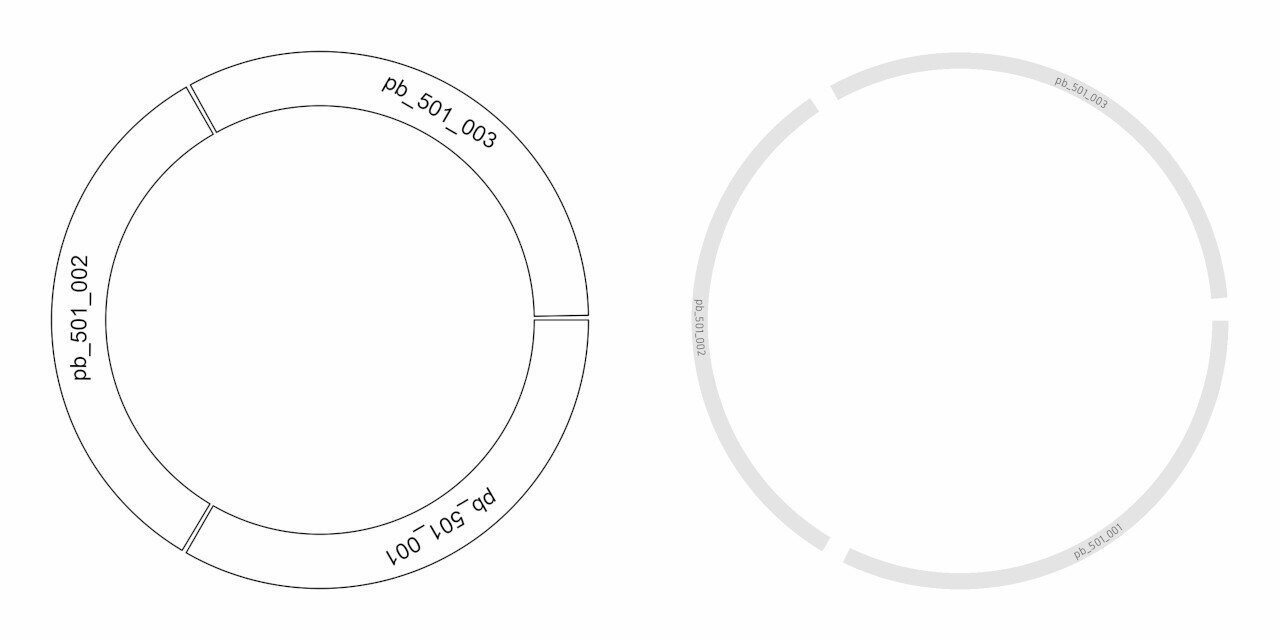Genome backbone seen using default setting (left) and customised settings (right).

Let’s add an x-axis to the plot:

# genomes x axis
circos.track(track.index = get.current.track.index(), panel.fun = function(x, y) {
circos.axis(h="top")
})

We can see the x-axis added (see below). We are going to add custom breaks, change label colour, size, direction and thickness of the axis line.

# genomes x axis
brk <- c(0, 0.5, 1, 1.5, 2, 2.5, 3, 3.5, 4, 4.5, 5)*10^6
circos.track(track.index = get.current.track.index(), panel.fun=function(x, y) {
circos.axis(h="top", major.at=brk, labels=round(brk/10^6, 1), labels.cex=0.4,
col=col_text, labels.col=col_text, lwd=0.7, labels.facing="clockwise")
}, bg.border=F)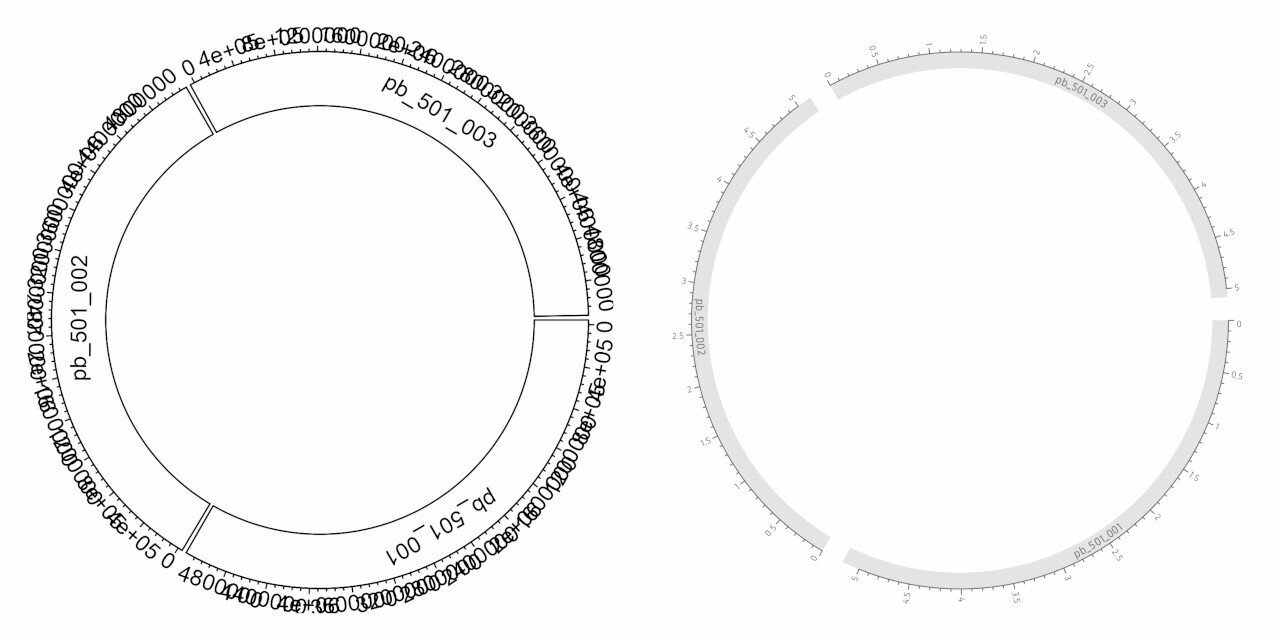Genome backbone with x-axis seen using default setting (left) and customised settings (right).

Coverage

Let’s add the coverage information and a y-axis for the coverage:

# coverage
circos.genomicTrack(data=cov, panel.fun=function(region, value, ...) {
circos.genomicLines(region, value)
})
# coverage y axis
circos.yaxis()

The coverage along the genome is displayed as a line. The y-axis is used to show a reference for the values that are plotted. We will change properties of the line such as colour and thickness. We add extra segments to act as gridlines. And we remove all y-axis elements except the text.

# coverage
circos.genomicTrack(data=cov, panel.fun=function(region, value, ...) {
circos.genomicLines(region, value, type="l", col="grey50", lwd=0.6)
circos.segments(x0=0, x1=max(ref\$V2), y0=100, y1=100, lwd=0.6, lty="11", col="grey90")
circos.segments(x0=0, x1=max(ref\$V2), y0=300, y1=300, lwd=0.6, lty="11", col="grey90")
#circos.segments(x0=0, x1=max(ref\$V2), y0=500, y1=500, lwd=0.6, lty="11", col="grey90")
}, track.height=0.08, bg.border=F)
circos.yaxis(at=c(100, 300), labels.cex=0.25, lwd=0, tick.length=0, labels.col=col_text, col="#FFFFFF")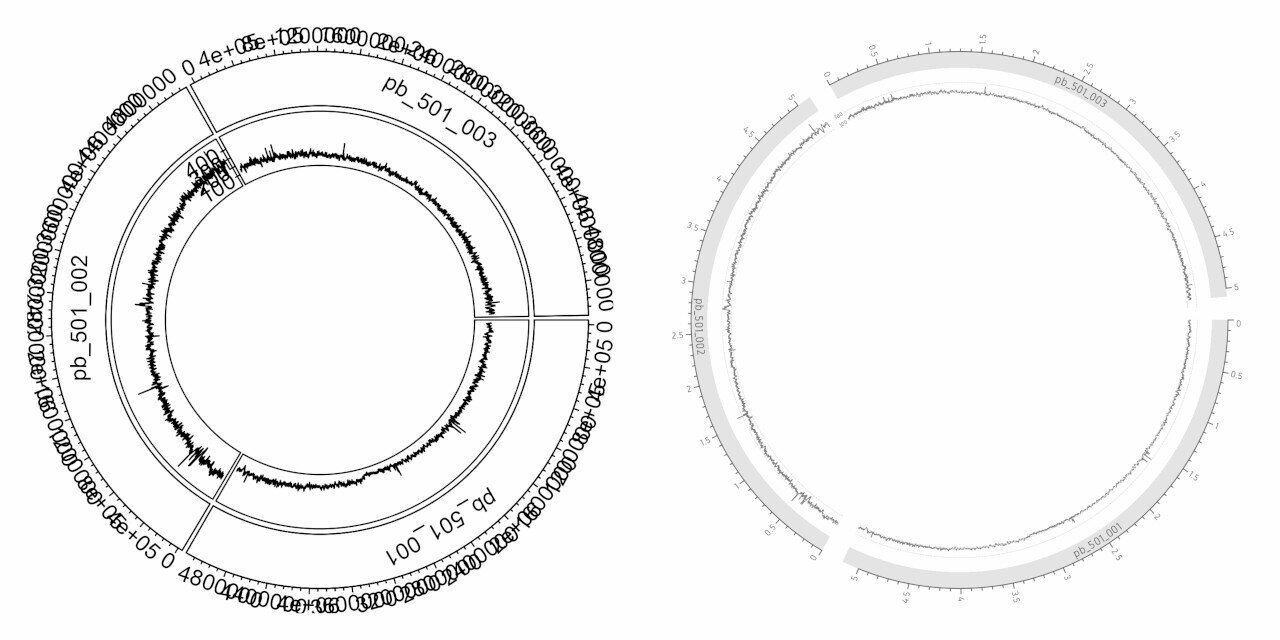Circlize plot showing coverage using default setting (left) and customised settings (right).

GC content

Let’s add GC content and it’s y-axis:

# gc content
circos.track(factors=gc\$chr, x=gc\$x, y=gc\$value, panel.fun=function(x, y) {
circos.lines(x, y)
})
# gc y axis
circos.yaxis()

Similar to the previous step, GC content is displayed as lines. The customisation is similar to the previous step. The gridline positions and values have obviously changed.

# gc content
circos.track(factors=gc\$chr, x=gc\$x, y=gc\$value, panel.fun=function(x, y) {
circos.lines(x, y, col="grey50", lwd=0.6)
circos.segments(x0=0, x1=max(ref\$V2), y0=0.3, y1=0.3, lwd=0.6, lty="11", col="grey90")
circos.segments(x0=0, x1=max(ref\$V2), y0=0.5, y1=0.5, lwd=0.6, lty="11", col="grey90")
circos.segments(x0=0, x1=max(ref\$V2), y0=0.7, y1=0.7, lwd=0.6, lty="11", col="grey90")
}, ylim=range(gc\$value), track.height=0.08, bg.border=F)
# gc y axis
circos.yaxis(at=c(0.3, 0.5, 0.7), labels.cex=0.25, lwd=0, tick.length=0, labels.col=col_text, col="#FFFFFF")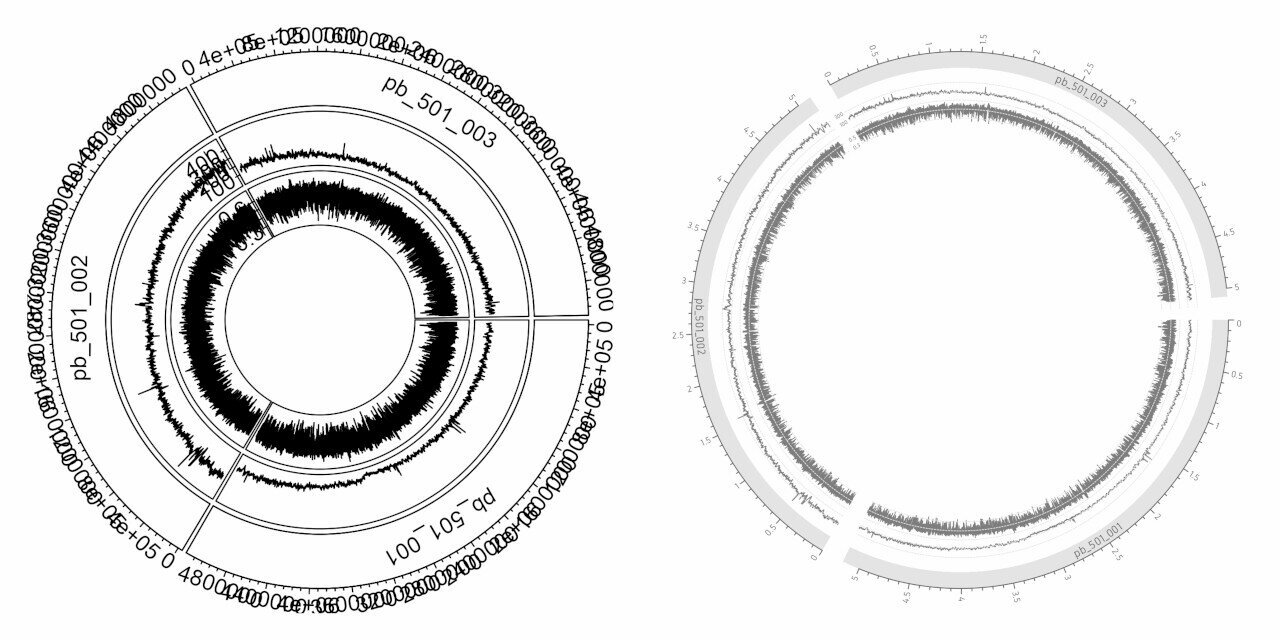Circlize plot showing GC content graph using default setting (left) and customised settings (right).

Gene labels

# gene labels
circos.genomicLabels(ann, labels.column=5)

The gene labels are added along with connection lines to indicate their exact position in the genome. This is too crowded without some manual customisation. We adjust the label size, colour and the length, thickness and colour of the connection lines. The track height is set to a custom value.

# gene labels
circos.genomicLabels(ann, labels.column=5, cex=0.25, col=col_text, line_lwd=0.5, line_col="grey80",
side="inside", connection_height=0.05, labels_height=0.04)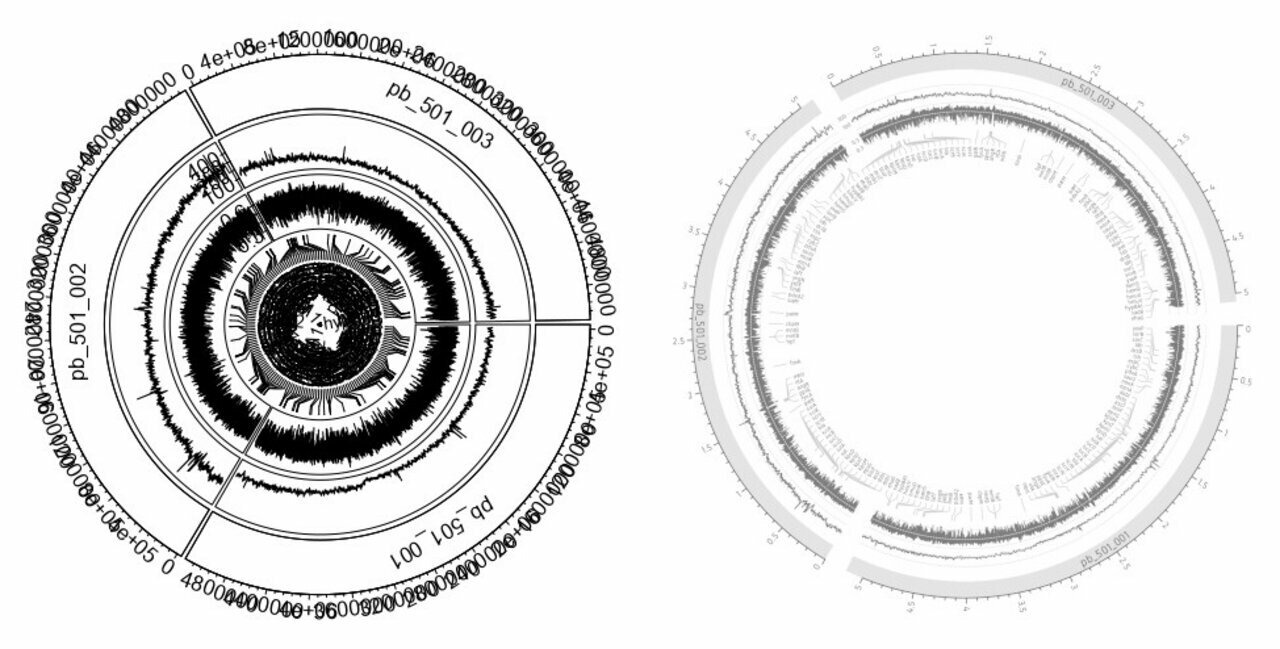Circlize plot showing gene positions and gene labels using default setting (left) and customised settings (right).

Rearrangements

# rearrangements

If you are running these steps interactively, this may not be visible as there is no space. But, if you run this inside a png() device, you can at least see it. Since there is no transparency, overlapping ribbons are not visible. We will assign green colour to rearrangements in the same direction and red colour to inversions. And we set 0.4 opacity for better visibility of overlapping ribbons.

# rearrangements
rcols <- scales::alpha(ifelse(sign(nuc1\$start - nuc1\$end) != sign(nuc2\$start - nuc2\$end), "#f46d43", "#66c2a5"), alpha=0.4)

And here we have the complete plot. Track heights for every track has been reduced to leave space for the rearrangement link figure in the middle.

For the purpose of captioning, I have further added some numbers in an image editing software. This will be useful to describe each part of the figure.

Roy Francis
Fast LD computation from VCF files using Tomahawk2018-12-01T13:45:07+01:002018-12-01T13:45:07+01:00https://royfrancis.com/fast-ld-computation-from-vcf-files-using-tomahawkInstall tomahawk from here. Following commands are run on the Linux command-line.

Thinning VCF

For LD computation, pairwise comparisons are performed between all SNPs, therefore it is essential to thin down the number of SNPs. My dataset has about 18 million SNPs. My aim is to bring it down to 20,000 or so. Use vcftools to thin down a VCF file.

vcftools --vcf snp.vcf --recode --recode-INFO-all --thin 50000 --out "snp-thin"

VCFtools - 0.1.15
(C) Adam Auton and Anthony Marcketta 2009

Parameters as interpreted:
--vcf snp.vcf
--recode-INFO-all
--thin 50000
--out snp-thin
--recode

After filtering, kept 64 out of 64 Individuals
Outputting VCF file...
After filtering, kept 26205 out of a possible 18793942 Sites
Run Time = 135.00 seconds

mv "snp-thin.recode.vcf" "snp-thin.vcf"

The argument --thin 50000 means to keep 1 SNP every 50,000 bases. Depending on the number of SNPs in the VCF file, you want to increase or decrease this. I am aiming for about 20,000 or so SNPs in the thinned file.

VCF to BCF

Convert VCF to BCF.

bcftools view snp.vcf -O b -o snp.bcf

Tomahawk

Instructions on using tomahawk is detailed here. Convert BCF to the tomahawk format. Import filters filter out sites with missingness > 20% and HW p-value < 0.001.

tomahawk import -i snp.bcf -o snp -m 0.2 -h 0.001

Tomahawk is used to compute LD.

Arguments: -u (unphased), -d (progress), -f (fast computation), -i (input file), -o (output file name prefix), -r (min R2 cut-off). See tomahawk help for more descriptions.

tomahawk calc -udfi snp.twk -o snp -a 5 -r 0.1 -P 0.1 -C 1 -t 1

The .two output is binary and needs to be exported to a text file. The -I arguments restricts the output to a specified chromosome. So, -I chr1 (UCSC) of -I 1 (Ensembl) exports only chromosome 1. It is probably better to have separate files for each chromosome as the files are a more managable size to read into R. The LD metrics are computed between SNPs within chromosomes anyway.

tomahawk view -i snp.two > snp.ld
tomahawk view -i snp.two -I 1 > snp-1.ld

Plotting

Now we move into R. First we load some libraries and define some plotting functions to be used later.

library(dplyr)
library(ggplot2)

# define plotting functions
#' @title plotPairwiseLD
#' @description Plots R2 heatmap across the chromosome (like Haploview)
#' @param dfr A data.frame with minimum CHROM_A, POS_A, CHROM_B, POS_B and R2.
#' An output from tomahawk works.
#' @param chr A chromosome name.
#' @param xlim A two number vector specifying min and max x-axis limits. Any one or both can be defaulted by specifying NA.
#' @param ylim A two number vector specifying min and max y-axis limits. Any one or both can be defaulted by specifying NA.
#' @param minr2 A value between 0 and 1. All SNPs with R2 value below this
#' value is excluded from plot.
#'
plotPairwiseLD <- function(dfr, chr, xlim = c(NA, NA), ylim = c(NA, NA), minr2) {
if (missing(dfr)) stop("Input data.frame 'dfr' missing.")

if (!missing(chr)) {
ld <- filter(ld, CHROM_A == get("chr") & CHROM_B == get("chr"))
}
ld <- filter(ld, POS_A < POS_B)

if (!missing(minr2)) {
ld <- filter(ld, R2 > get("minr2"))
}

ld <- ld %>% arrange(R2)

ld\$x <- ld\$POS_A + ((ld\$POS_B - ld\$POS_A) / 2)
ld\$y <- ld\$POS_B - ld\$POS_A
ld\$r2c <- cut(ld\$R2, breaks = seq(0, 1, 0.2), labels = c(
"0-0 - 0.2", "0.2 - 0.4",
"0.4 - 0.6", "0.6 - 0.8",
"0.8 - 1.0"
))
ld\$r2c <- factor(ld\$r2c, levels = rev(c(
"0-0 - 0.2", "0.2 - 0.4",
"0.4 - 0.6", "0.6 - 0.8",
"0.8 - 1.0"
)))

ggplot(ld, aes(x = x, y = y, col = r2c)) +
geom_point(shape = 20, size = 0.1, alpha = 0.8) +
scale_color_manual(values = c("#ca0020", "#f4a582", "#d1e5f0", "#67a9cf", "#2166ac")) +
scale_x_continuous(limits = xlim) +
scale_y_continuous(limits = ylim) +
guides(colour = guide_legend(title = "R2", override.aes = list(shape = 20, size = 8))) +
labs(x = "Chromosome (Bases)", y = "") +
theme_bw(base_size = 14) +
theme(
panel.border = element_blank(),
axis.ticks = element_blank()
) %>%
return()
}

#' @title plotDecayLD
#' @description Plots R2 heatmap across the chromosome (like Haploview)
#' @param dfr A data.frame with minimum CHROM_A, POS_A, CHROM_B, POS_B and R2.
#' An output from tomahawk works.
#' @param chr A chromosome name.
#' @param xlim A two number vector specifying min and max x-axis limits. Any one or both can be defaulted by specifying NA.
#' @param ylim A two number vector specifying min and max y-axis limits.  Any one or both can be defaulted by specifying NA.
#' @param avgwin An integer specifying window size. Mean R2 is computed within windows.
#' @param minr2 A value between 0 and 1. All SNPs with R2 value below this
#' value is excluded from plot.
#'
plotDecayLD <- function(dfr, chr, xlim = c(NA, NA), ylim = c(NA, NA), avgwin = 0, minr2) {
if (missing(dfr)) stop("Input data.frame 'dfr' missing.")

if (!missing(chr)) {
ld <- filter(ld, CHROM_A == get("chr") & CHROM_B == get("chr"))
}
ld <- filter(ld, POS_A < POS_B)

if (!missing(minr2)) {
ld <- filter(ld, R2 > get("minr2"))
}

ld <- ld %>% arrange(R2)

ld\$dist <- ld\$POS_B - ld\$POS_A

if (avgwin > 0) {
ld\$distc <- cut(ld\$dist, breaks = seq(from = min(ld\$dist), to = max(ld\$dist), by = avgwin))
ld <- ld %>%
group_by(distc) %>%
summarise(dist = mean(dist), R2 = mean(R2))
}

ggplot(ld, aes(x = dist, y = R2)) +
geom_point(shape = 20, size = 0.15, alpha = 0.7) +
geom_smooth() +
scale_x_continuous(limits = xlim) +
scale_y_continuous(limits = ylim) +
labs(x = "Distance (Bases)", y = expression(LD ~ (r^{
2
}))) +
theme_bw(base_size = 14) +
theme(
panel.border = element_blank(),
axis.ticks = element_blank()
) %>%
return()
}

The output looks like below:

FLAG CHROM_A   POS_A CHROM_B   POS_B   REF_REF      REF_ALT   ALT_REF
1   10       1     359       1  100777 103.00000 8.526513e-14  5.000000
2   10       1  100777       1     359 103.00000 8.526513e-14  5.000000
3    2       1     359       1  452615  97.48765 5.512354e+00 10.512354
4    2       1  452615       1     359  97.48765 5.512354e+00 10.512354
5    6       1     359       1 1454410  49.07769 5.392231e+01  1.922308
6    6       1 1454410       1     359  49.07769 5.392231e+01  1.922308
ALT_ALT          D    Dprime         R        R2            P ChiSqModel
1 20.00000 0.12573242 1.0000000 0.8734775 0.7629629 4.440203e-19   2.296495
2 20.00000 0.12573242 1.0000000 0.8734775 0.7629629 4.440203e-19   2.296495
3 14.48765 0.08266716 0.6574848 0.5742982 0.3298184 5.573234e-08   1.993300
4 14.48765 0.08266716 0.6574848 0.5742982 0.3298184 5.573234e-08   1.993300
5 23.07769 0.06280179 0.8070154 0.3235735 0.1046998 1.876711e-04   7.703488
6 23.07769 0.06280179 0.8070154 0.3235735 0.1046998 1.876711e-04   7.703488
ChiSqTable
1   97.65926
2   97.65926
3   42.21675
4   42.21675
5   13.40157
6   13.40157

Plot pairwise LD plot.

plotPairwiseLD(ld)
plotPairwiseLD(ld, minr2=0.2)
plotPairwiseLD(ld, minr2=0.2, ylim=c(0, 10^7))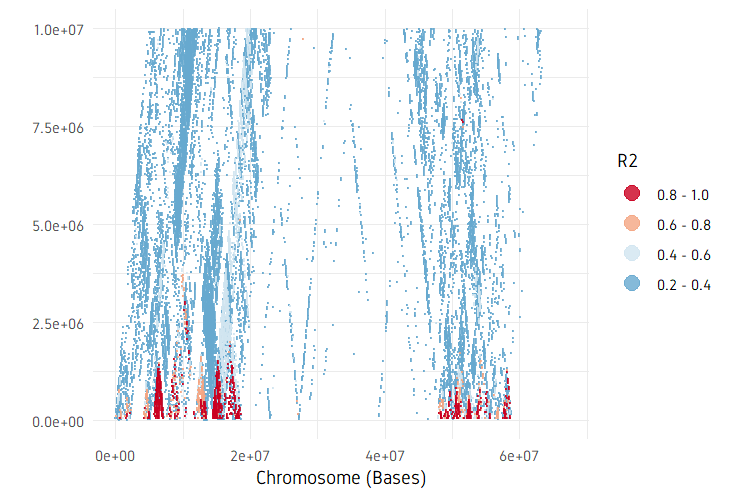Pairwise LD plot with a min R2 limit of 0.2 and y-axis max limit set to 10^7.
plotDecayLD(ld, minr2=0.25)
plotDecayLD(ld, minr2=0.25, avgwin=100000)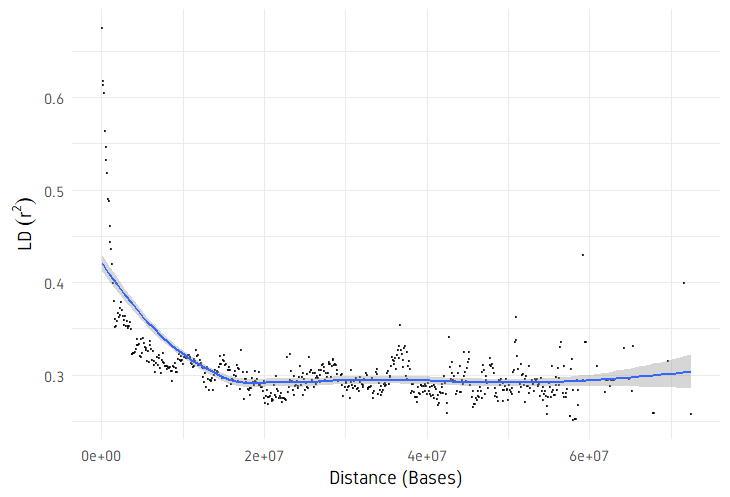LD decay plot with a min R2 limit of 0.25 and R2 values averaged within 10 Kb windows. ]]>
Roy Francis
Using google maps for aerial photography2018-08-20T21:40:09+02:002018-08-20T21:40:09+02:00https://royfrancis.com/using-google-maps-for-aerial-photographyIf you are into aerial photography, especially using a drone, then you know that it’s not easy to imagine how the world looks like from above. Composition from the air adds an extra dimension to usual level of photo composition difficulty. The most obvious choice would be to find a high point (hill, building etc) to get a sense of the landscape. When that is not good enough, we have Google Earth. Now for those of you who were paying attention to google maps, you might have noticed that google maps is now available in 3D. At least in some areas and major cities. For those areas, that are available, the level of detail is stunning. The views are far superior than the blocky 3D seen previously in Google Earth. I mean, just look at this:

This is so impressive. It looks like an open-world game. Every tree is rendered independently and probably has several thousand polygons. Now with 3D control, I can survey the area I want to photograph and compose my scene just the way I want. To show you some examples, below are some of the scenes composed in google maps and then actually photographed using a drone.

This is brilliant because I don’t need to use up a whole battery on my drone just to find a composition. The limitation is that this detailed 3D model is not available everywhere and also does not reflect the current lighting and weather conditions. It would also be great if google maps showed us the height of the viewpoint/camera when in 3D. Another useful additional would be to somehow incorporate SunCalc into this to get the position and trajectory of the sun.

]]>
Roy Francis
Calendar plot shiny app and dynamic UI2018-08-12T22:10:52+02:002018-08-12T22:10:52+02:00https://royfrancis.com/calendar-plot-shiny-app-and-dynamic-uiIn my previous post, I created a calendar planner plot using ggplot2. I wanted to take it further and create a shiny app for it. This was more of a shiny learning exercise rather than a purpose-driven app. Specifically, creating dynamic user inputs and retrieving data from these inputs.

The app looks like this:

So I had my core plotting code from the previous post, and then I created user interfaces around it. The layout is simple; there is a panel on the left with input widgets and the panel on the right displays the plot. The app takes in user input for a date span (start and end duration) and a data.frame is created expanding that span to a sequence of dates.

I wanted the start date duration and end date duration widgets to be side-by-side in one row, and therefore, I have used custom divs. For date input, I have set the default start date to be today’s date and end date to be 30 days from today.

div(class="row",
div(class="col-md-6", dateInput("in_duration_date_start", "From", value=format(as.Date(Sys.time(), "%Y-%m-%d", tz="Europe/Stockholm"), "%Y-%m-%d"))),
div(class="col-md-6", dateInput("in_duration_date_end","To", value=format(as.Date(Sys.time(), "%Y-%m-%d", tz="Europe/Stockholm")+30, "%Y-%m-%d")))
)

Then the user adds more date spans to define various activities. I call these tracks. Rather than setting up a pre-defined number of tracks, I wanted to let the user choose the number of tracks. And each track will have some track parameters. I have defined a track name, start date, end date and a colour for the track. This called for a bit more complex code. The track inputs are dynamically created in a loop depending on the number of tracks set by the user.

The code that generates the track input user-interface (UI) dynamically looks like this:

output\$tracks <- renderUI({
num_tracks <- as.integer(input\$in_num_tracks)

# create date intervals
dseq <- seq(as.Date(input\$in_duration_date_start), as.Date(input\$in_duration_date_end), by=1)
r1 <- unique(as.character(cut(dseq, breaks=num_tracks+1)))

lapply(1:num_tracks, function(i) {

div(class="row",
div(class="col-xs-3", style=list("padding-right: 3px;"), textInput(paste0("in_track_name_", i), label="Name", value=paste0("Text", i), placeholder="Available")),
div(class="col-xs-3", style=list("padding-left: 3px;"), colourpicker::colourInput(paste0("in_track_colour_", i), label="Colour", palette="limited", allowedCols=cols, value=cols[i]))
)
})
})

This looks like this in action:

Based on the number of input tracks, the date duration span is split into equal intervals by default. The start and end dates of the intervals are used as the default spans for track dates. Default colours are also selected by looping through a pre-defined colour vector.

A core function takes in all the plot related input to create the plot and returns a ggplot2 object. This function is called by the renderImage() function that creates the preview plot and also by the download plot handler. Inside the core function, a loop is also used to read the input track parameters back. The relevant part of the code looks like this:

num_tracks <- input\$in_num_tracks
track_cols <- vector(length=num_tracks)
tracks <- vector(length=num_tracks)
for(i in 1:num_tracks)
{
temp_start_date <- as.Date(as.character(eval(parse(text=paste0("input\$in_track_date_start_", i)))))
temp_end_date <- as.Date(as.character(eval(parse(text=paste0("input\$in_track_date_end_", i)))))
temp_track_name <- as.character(eval(parse(text=paste0("input\$in_track_name_", i))))
temp_track_col <- as.character(eval(parse(text=paste0("input\$in_track_colour_", i))))
}

I have only focused on the exciting bit of code that is new to me. The full code is available at this GitHub repo. A demo of this app is available at this shinyapps.io link. This link may not always be active.

]]>
Roy Francis
Calendar plot with ggplot22018-08-04T09:28:30+02:002018-08-04T09:28:30+02:00https://royfrancis.com/calendar-plot-with-ggplot2So, someone asked me about my summer time availability at work. I realized that my availability was a bit complex and perhaps it was easier to sent a figure/diagram rather than explaining it in 200 words. That’s where I thought of the idea of creating an availability calendar using ggplot2. The idea is to show basically three categories: available, not available and a limited period (where I am not available in person, but can read emails).

First, we prepare the general date range as a data.frame. Character dates are converted to date format. Then day, week, month and date are extracted from that as separate columns for ease of plotting.

# prepare date range
dfr <- data.frame(date=seq(as.Date('2018-06-11'), as.Date('2018-08-12'), by=1))
dfr\$day <- factor(strftime(dfr\$date, format="%a"), levels=rev(c("Mon", "Tue", "Wed", "Thu", "Fri", "Sat", "Sun")))
dfr\$week <- factor(strftime(dfr\$date, format="%V"))
dfr\$month <- factor(strftime(dfr\$date, format="%B"), levels=c("June", "July", "August"))
dfr\$ddate <- factor(strftime(dfr\$date, format="%d"))

date day week month ddate
1 2018-06-11 Mon   24  June    11
2 2018-06-12 Tue   24  June    12
3 2018-06-13 Wed   24  June    13
4 2018-06-14 Thu   24  June    14
5 2018-06-15 Fri   24  June    15
6 2018-06-16 Sat   24  June    16

Then we add our tracks to define availability into a new column named comment. We first set all comments to “Available” and then overwrite the ranges based on our availability. I have also added weekend as a category so it can be coloured differently.

dfr\$comment <- "Available"
dfr\$comment[dfr\$date>=as.Date('2018-06-11') & dfr\$date<=as.Date('2018-06-20')] <- "Limited"
dfr\$comment[dfr\$date>=as.Date('2018-06-21') & dfr\$date<=as.Date('2018-06-29')] <- "Not available"
dfr\$comment[dfr\$date>=as.Date('2018-07-09') & dfr\$date<=as.Date('2018-07-13')] <- "Not available"
dfr\$comment[dfr\$date>=as.Date('2018-08-06') & dfr\$date<=as.Date('2018-08-10')] <- "Not available"
dfr\$comment[dfr\$day=="Sat" | dfr\$day=="Sun"] <- "Weekend"

Then I convert the comment column into a factor with custom level order. This order determines the order in which the labels will be shown in the plot legend.

dfr\$comment <- factor(dfr\$comment, levels=c("Available", "Limited", "Not available", "Weekend"))

Finally, the plotting. Columns week and day are used to define x and y axes. geom_tile() creates the blocks and colours for each day. facet_grid()  is used to separate months. scale_fill_manual() is used to assign custom colours. theme_bw() and theme() are used for fine adjustment of plot layout.

# plot
p <- ggplot(dfr, aes(x=week, y=day))+
geom_tile(aes(fill=comment))+
geom_text(aes(label=ddate))+
scale_fill_manual(values=c("#8dd3c7", "#ffffb3", "#fb8072", "#d3d3d3"))+
facet_grid(~month, scales="free", space="free")+
labs(x="Week", y="")+
theme_bw(base_size=10)+
theme(legend.title=element_blank(),
panel.grid=element_blank(),
panel.border=element_blank(),
axis.ticks=element_blank(),
strip.background=element_blank(),
legend.position="top",
legend.justification="right",
legend.direction="horizontal",
legend.key.size=unit(0.3, "cm"),
legend.spacing.x=unit(0.2, "cm"))

And then save the plot.

ggsave("calendar.png", p, height=10, width=14, units="cm", dpi=300, type="cairo")